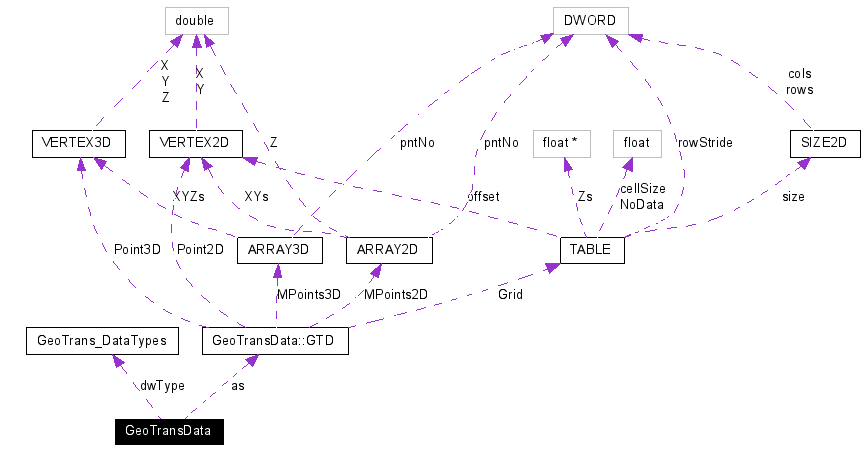﻿ GeoTransData structure

GeoTransData structure

Declared in: GeoTransformers.h

Self descriptive I/O package holding data interpreted by a GeoTransformer.

[C / C++]

typedef struct {

GeoTrans_DataTypes dwType; // describes package type

union GTD {

VERTEX3D Point3D; // single 3D point

VERTEX2D Point2D; // single 2D point

ARRAY3D MPoints3D; // multiple 3D points

ARRAY2D MPoints2D; // multiple 2D points

TABLE Grid; // Grid of float Z values

} * as; // pointer to data structure

} GeoTransData;

Collaboration diagram:Collaboration graph for GeoTransData structure

Members

dwType

It describes the type of I / O package interpreted by GeoTransformers. For details on defined values of this member, please refer to GeoTransDataTypes documentation.

as

Pointer to an encapsulated data structure as defined by the GeoTransData::GTD union. In order to access the correct structure as was allocated, below is the association table for defined types:

 GeoTransDataTypes GeoTransData::GTD dwType = geoData_Point3D Use as->Point3D member. Its structure type is VERTEX3D. dwType = geoData_Point2D Use as->Point2D member. Its structure type is VERTEX2D. dwType = geoData_MPoints3D Use as->MPoints3D member. Its structure type is ARRAY3D. dwType = geoData_MPoints2D Use as->MPoints2D member. Its structure type is ARRAY2D. dwType = geoData_Grid Use as->Grid member. Its structure type is TABLE.

Remarks

Your implementation should remain compatible with later versions of GeoTransCore component, which might introduce new types of packages to GeoTransDataTypes. Both transformation functions test their input and output as explained in their documentation's Remarks section.

Requirements

 Platform 32 bit Windows OS Environment ANSI C / C++ Standard compliant

Example

Following sample code shows how to process packages containing arrays of 3D / 2D points:

[C / C++]

if (inData.dwType & 0x2) { // arrays given

if (inData.as->MPoints2D.pntNo != outData.as->MPoints2D.pntNo) {

SetLastError(err_data_pnts_dif);

return FALSE;

}

int iStride = 3-(inData.dwType & 0x1); // Stride2D=2;Stride3D=3

int oStride = 3-(outData.dwType & 0x1); // Stride2D=2;Stride3D=3

register int idx = inData.as->MPoints2D.pntNo; // same offset in MPoints3D ;)

double * iXYs = (double*)inData.as->MPoints2D.XYs; // same offset in MPoints3D ;)

double * oXYs = (double*)outData.as->MPoints2D.XYs; // same offset in MPoints3D ;)

while (idx) { --idx;

VERTEX3D& ip = *(VERTEX3D*)iXYs;

VERTEX3D& op = *(VERTEX3D*)oXYs;

double iZ; // input Z

if (inData.dwType & 0x1) { // 2D array that shares same Z

iZ = inData.as->MPoints2D.Z;

}

else { // 3D array

iZ = ip.Z;

}

double * poZ; // output Z

if (outData.dwType & 0x1) { // 2D array that shares same Z

poZ = &outData.as->MPoints2D.Z;

}

else { // 3D array

poZ = &op.Z;

}

/* TODO: process (ip.X,ip.Y,iZ) and store result to (op.X,op.Y,*poZ) */

iXYs += iStride;

oXYs += oStride;

}

return TRUE;

}

For a detailed example using this structure, please see Custom GeoTransformer VC++ Sample.

Related Topics

Referenced By: GeoTrans_FromGlobalDirection, GeoTrans_ToGlobalDirection

References: GeoTransDataTypes, GeoTransData::GTD